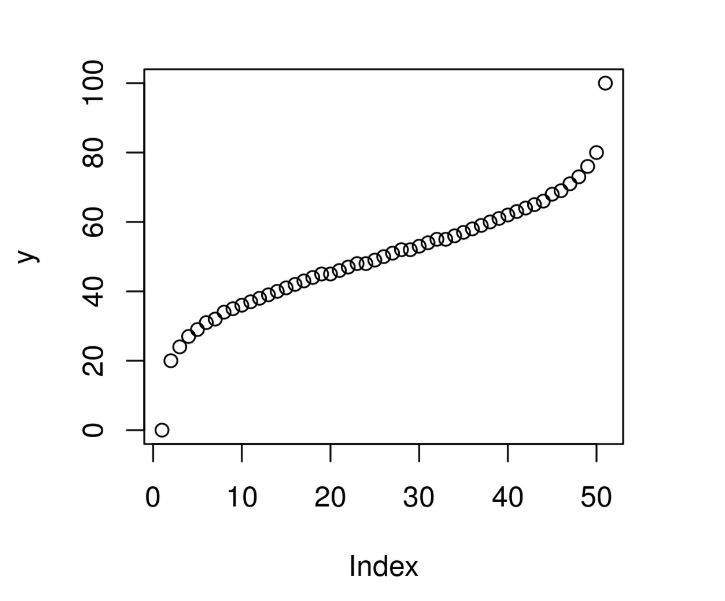Open in App
Not now

# Compute the value of Quantile Function over Wilcoxon Rank Sum Distribution in R Programming – qwilcox() Function

• Last Updated : 25 Jun, 2020

`qwilcox()` function in R Language is used to compute the value of Quantile Function(QF) for Wilcoxonrank Sum Statistic Distribution over a sequence of Numeric Values.

Syntax: qwilcox(x, m, n)

Parameters:
x: Numeric Vector
m: Larger sample size
n: Smaller sample size

Example 1:

 `# R Program to compute the value of``# QF over Wilcoxonrank Sum Distribution`` ` `# Creating a sequence of x-values``x <``-` `seq(``0``, ``1``, by ``=` `0.1``)`` ` `# Calling qwilcox() Function``y <``-` `qwilcox(x, m ``=` `10``, n ``=` `5``)``y`

Output:

```   0 14 18 21 23 25 27 29 32 36 50
```

Example 2:

 `# R Program to compute the value of``# QF over Wilcoxonrank Sum Distribution`` ` `# Creating a sequence of x-values``x <``-` `seq(``0``, ``1``, by ``=` `0.02``)`` ` `# Calling qwilcox() Function``y <``-` `qwilcox(x, m ``=` `20``, n ``=` `5``)`` ` `# Plot a graph``plot(y)`

Output:My Personal Notes arrow_drop_up